# What is the difference between parseInt() and Number()?

• The parseInt() function:
The parseInt() function is used to parse a string and convert it to an integer of a specified radix. It takes two parameters, the string to be parsed and the radix to be used. The radix is an integer between 2 and 36 which represents the base of the number.

If parseInt() encounters a character while parsing that does not conform to the specified radix, it will ignore the character and all succeeding characters. It then returns the value parsed up to that point as an integer. Spaces that are leading or trailing are allowed in this case.

If the function gets the first character and cannot convert it to a number, it will return NaN unless the radix is bigger than 10. This NaN value is not a valid number for any radix and cannot be used in any mathematical calculation.

Syntax:

`parseInt(string, radix)`
• The Number() function
The Number() function is used to create a primitive type Number object. It takes one parameter which is the value of the number. This value could be passed with a string and the Number function will try to represent it as a number. If the argument could not be converted into a number, it returns a NaN value. This NaN value is not a valid number and cannot be used in any mathematical calculation.

Syntax:

`Number(valueString)`

The differences between these can be explained using these below examples:

Example 1:
This example shows that parseInt() tries to convert the value to the last character that could be converted to an integer. The trailing whitespaces and characters are ignored as they are not valid. The Number() function on the other hand just returns NaN.

 ` ` `<``html``> ` ` `  `<``head``> ` `    ``<``title``> ` `      ``What is the difference between  ` `      ``parseInt() and Number() ` `  `` ` ` ` ` `  `<``body``> ` `    ``<``h1` `style``=``"color: green"``> ` `      ``GeeksforGeeks ` `  `` ` `    ``<``b``> ` `      ``What is the difference between ` `      ``parseInt() and Number() ` `  `` ` `    ``<``p``>String is: 10 objects ` `    ``<``p``>Output of parseInt is: <``span` `class``=``"parseOutput"``> ` `      `` ` `  `` ` `    ``<``p``>Output of Number is: <``span` `class``=``"numberOutput"``> ` `      `` ` `  `` ` `    ``<``button` `onclick``=``"parseNumber()"``> ` `      ``Parse string ` `  `` ` `    ``<``script` `type``=``"text/javascript"``> ` `        ``function parseNumber() { ` `            ``let string = '10.6 objects'; ` `            ``let number1 = parseInt(string); ` `            ``let number2 = Number(string); ` ` `  `            ``document.querySelector( ` `              ``'.parseOutput').textContent = number1; ` `            ``document.querySelector( ` `              ``'.numberOutput').textContent = number2; ` `        ``} ` `    `` ` ` ` ` `  ` `

Output:

• Before clicking the button: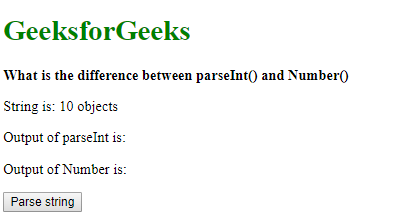• After clicking the button: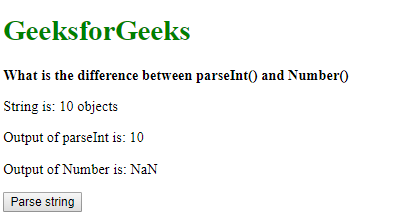Example 2:
This example shows the difference that parseInt() only returns an integer value whereas Number() returns all the digits including floating points.

 ` ` `<``html``> ` ` `  `<``head``> ` `    ``<``title``> ` `      ``What is the difference between  ` `      ``parseInt() and Number() ` `  `` ` ` ` ` `  `<``body``> ` `    ``<``h1` `style``=``"color: green"``> ` `      ``GeeksforGeeks ` `  `` ` `    ``<``b``>What is the difference between ` `      ``parseInt() and Number() ` `  `` ` `    ``<``p``>String is: 3.1415 ` `    ``<``p``>Output of parseInt is: <``span` `class``=``"parseOutput"``> ` `      `` ` `  `` ` `    ``<``p``>Output of Number is: <``span` `class``=``"numberOutput"``> ` `      `` ` `  `` ` `    ``<``button` `onclick``=``"parseNumber()"``>Parse string ` `    ``<``script` `type``=``"text/javascript"``> ` `        ``function parseNumber() { ` `            ``let string = '3.1415'; ` `            ``let number1 = parseInt(string); ` `            ``let number2 = Number(string); ` ` `  `            ``document.querySelector( ` `              ``'.parseOutput').textContent = number1; ` `            ``document.querySelector( ` `              ``'.numberOutput').textContent = number2; ` `        ``} ` `    `` ` ` ` ` `  ` `

Output:

• Before clicking the button: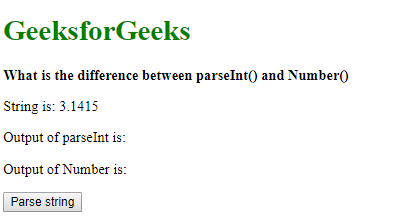• After clicking the button: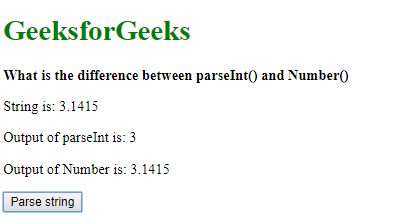Example 3:
This example shows the working of the radix parameter in parseInt(). The string passed is parsed with the base of 2. This returns the value as 12. On the other hand, Number() returns the value in the string as-is.

 ` ` `<``html``> ` ` `  `<``head``> ` `    ``<``title``> ` `      ``What is the difference between  ` `      ``parseInt() and Number() ` `  `` ` ` ` ` `  `<``body``> ` `    ``<``h1` `style``=``"color: green"``> ` `      ``GeeksforGeeks ` `  `` ` `    ``<``b``>What is the difference between ` `      ``parseInt() and Number() ` `  `` ` `    ``<``p``>String is: 1100 ` `    ``<``p``>Output of parseInt is: <``span` `class``=``"parseOutput"``> ` `     `  `      `` ` `    ``<``p``>Output of Number is: <``span` `class``=``"numberOutput"``> ` `      `` ` `    ``<``button` `onclick``=``"parseNumber()"``> ` `      ``Parse string ` `  `` ` `   `  `    ``<``script` `type``=``"text/javascript"``> ` `        ``function parseNumber() { ` `            ``let string = '1100'; ` `            ``let number1 = parseInt(string, 2); ` `            ``let number2 = Number(string); ` ` `  `            ``document.querySelector( ` `              ``'.parseOutput').textContent = number1; ` `            ``document.querySelector( ` `              ``'.numberOutput').textContent = number2; ` `        ``} ` `    `` ` ` ` ` `  ` `

Output:

• Before clicking the button: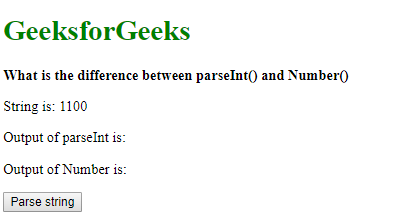• After clicking the button: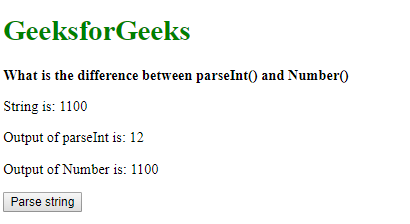My Personal Notes arrow_drop_upIm listening

If you like GeeksforGeeks and would like to contribute, you can also write an article using contribute.geeksforgeeks.org or mail your article to contribute@geeksforgeeks.org. See your article appearing on the GeeksforGeeks main page and help other Geeks.

Please Improve this article if you find anything incorrect by clicking on the "Improve Article" button below.